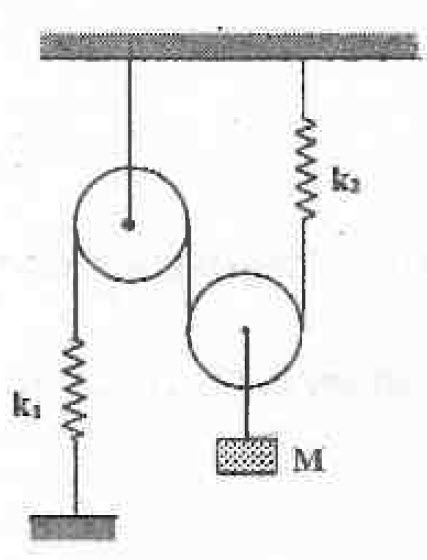# Pull, and let goFind the Time Period of oscillations (in $\displaystyle \text{sec}$) for the arrangement as shown in the figure.

Details and Assumptions:

• The pulleys are smooth and massless.
• $\displaystyle k_1 = 100 N/m$
• $\displaystyle k_2 = 200 N/m$
• $\displaystyle M = 4 kg$
• $\displaystyle g = 9.8 m/s^2$
×

Problem Loading...

Note Loading...

Set Loading...